# Lap Winding: Simplex and Duplex Lap Windings (Formula & Diagrams)

Contents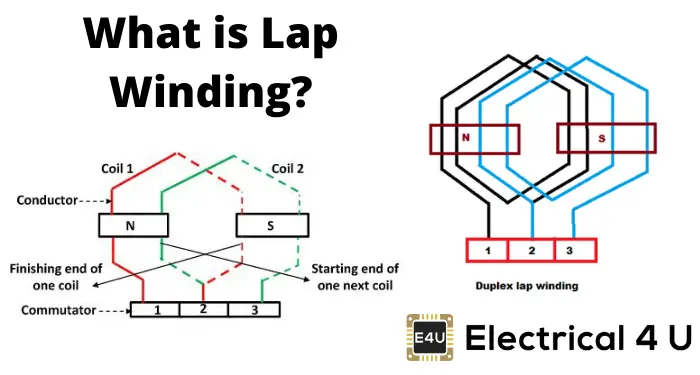Armature windings can be classified into two types – lap windings and wave windings. Here we are going to discuss lap windings and the two main types of lap windings in DC machines.

These are known as Simplex Lap Windings and Duplex Lap Windings.

## What is a Lap Winding?

A lap winding is a winding in which successive coils overlap each other. It is named a “Lap” winding because it doubles or laps back with its succeeding coils. A diagram of a lap winding configuration is shown below.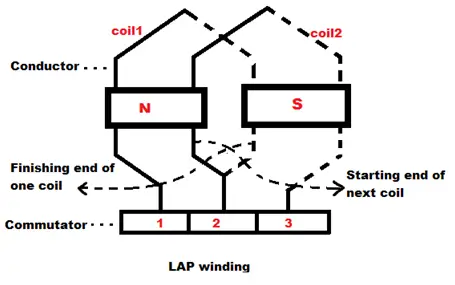In this winding, the finishing end of one coil is connected to one commutator segment, and the starting end of the next coil situated under the same pole and connected with the same commutator segment.

Here we can see in the picture, the finishing end of coil – 1 and the starting end of coil – 2 are both connected to the commutator segment – 2 and both coils are under the same magnetic pole that is the N pole here.

There are two different types of Lap Windings:

1. Simplex Lap Winding
2. Duplex Lap Winding

## Simplex Lap Winding

A winding in which the number of parallel path between the brushes is equal to the number of poles is called simplex lap winding.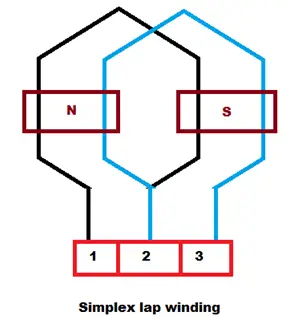## Duplex Lap Winding

A winding in which the number of the parallel paths between the brushes is twice the number of poles is called duplex lap winding.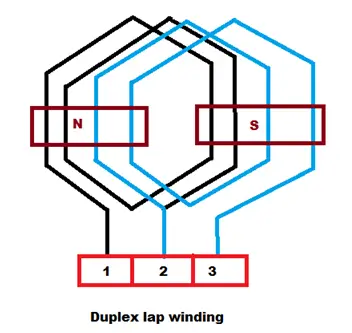Some important points to remember while designing the Lap winding:
If,

• Z = the number of conductors
• P = number of poles
• YB = Back pitch
• YF = Front pitch
• YC = Commutator pitch
• YA = Average pole pitch
• YP = Pole pitch
• YR = Resultant pitch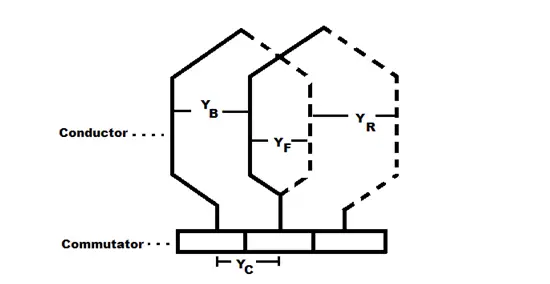Then, the back and front pitches are of opposite sign and they cannot be equal.
YB = YF ± 2m

• m = multiplicity of the winding.
• m = 1 for Simplex Lap winding
• m = 2 for Duplex Lap winding

When,
YB > YF, it is called progressive winding.
YB < YF, it is called retrogressive winding.

The back pitch and front pitch must be odd.

Resultant pitch (YR) = YB – YF = 2m

YR is even because it is the difference between two odd numbers.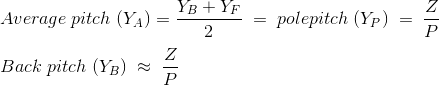Commutator pitch (YC) = ±m
Number of parallel path in the Lap winding = mP
Let us start from 1st conductor,

 Back connections Front connections 1 to (1+YB) = (1+5) = 6 6 to (6-YF) = (6 – 3) = 3 3 to (3+5) = 8 8 to (8-3) = 5 5 to (5+5) = 10 10 to (10-3) = 7 7 to (7+5) = 12 12 to (12-3) = 9 9 to (9+5) = 14 14 to (14-3) = 11 11 to (11+5) = 16 16 to (16-3) =13 13 to (13+5) = 18 = (18-16) = 2 2 to (18-3) = 15 15 to (15+5) = 20 = (20-16) = 4 4 to (20-3) = 17 = (17-16) = 1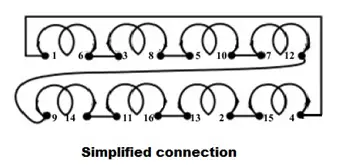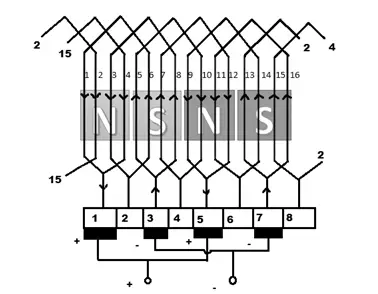The advantages of lap windings include:

1. This winding is necessarily required for large current applications because it has more parallel paths.
2. It is suitable for low voltage and high current generators.

The disadvantages of lap windings include:

1. It gives less emf compared to wave winding. This winding requires more no. of conductors for giving the same emf, which results in high winding cost.
2. It has less efficient utilization of space in the armature slots.

Want To Learn Faster? 🎓
Get electrical articles delivered to your inbox every week.
No credit card required—it’s 100% free.Electrical4U is dedicated to the teaching and sharing of all things related to electrical and electronics engineering.

### 2 thoughts on “Lap Winding: Simplex and Duplex Lap Windings (Formula & Diagrams)”

1.thank you sir ….easy to understand

•